Aa Bb Cc Dd Ee Ff Gg Hh Ii Jj Kk Ll Mm Nn Oo Pp Qq Rr Ss Tt Uu Vv Ww Xx Yy Zz

Rr

rate

• a ratio between two measurements using different units, for example,
births per year, cost per person, words per minute.

EXAMPLES: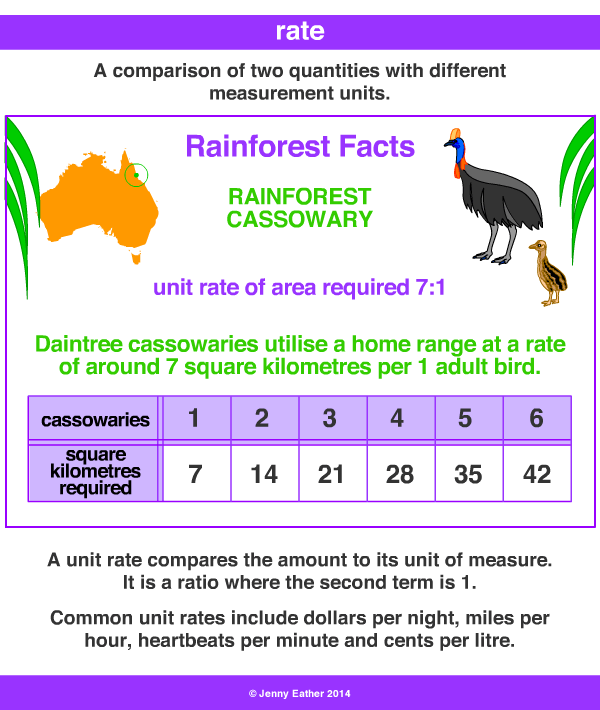interest rate

•  interest is expressed as a percentage rate
of the principal for a given time interval.
• the principal is the amount being borrowed.
• the interest is a fee paid for
the right to borrow.

simple interest formula

I = P x R x T

I = Interest, P = Principle, R = Rate, T = Time

EXAMPLE: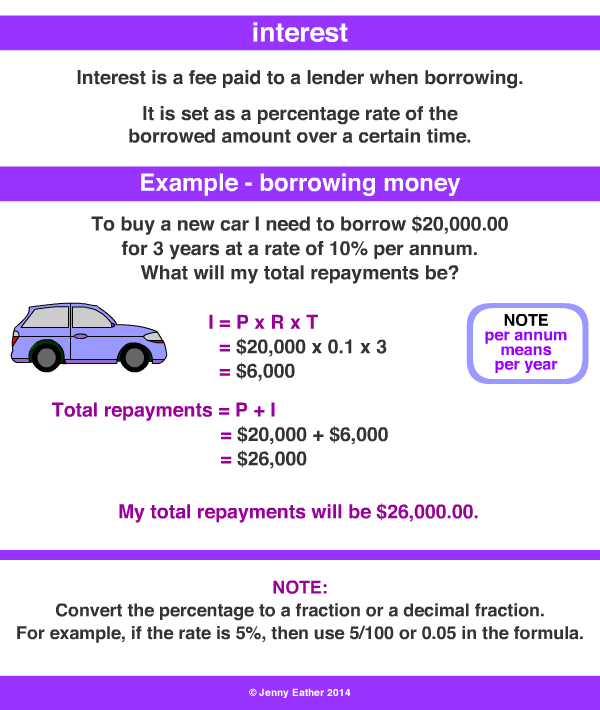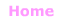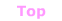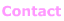© Jenny Eather 2014. All rights reserved.